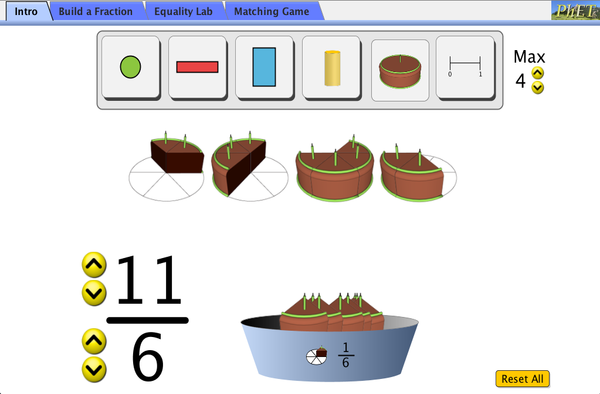# 分數的介紹下載嵌入 關閉 嵌入啓動模擬教學之圖樣返回 HTML5 版本

### 標題

• Fractions
• Equivalent Fractions
• Improper Fraction
• Number Line

### 描述

Amber Chang (2012)

HTML5 版本之翻譯：

### 學習目標

• Predict and explain how changing the numerator of a fraction affects the fraction's value
• Predict and explain how changing the denominator of a fraction affects the fraction's value
• Convert between a picture of a fraction, a numeric fraction, and a point on a number line
• Build matching fractions using numbers and pictures
• Compare fractions using numbers and patterns

### 標準對齊

#### 共用核心 - 數學

1.G.A.3
Partition circles and rectangles into two and four equal shares, describe the shares using the words halves, fourths, and quarters, and use the phrases half of, fourth of, and quarter of. Describe the whole as two of, or four of the shares. Understand for these examples that decomposing into more equal shares creates smaller shares.
2.G.A.2
Partition a rectangle into rows and columns of same-size squares and count to find the total number of them.
2.G.A.3
Partition circles and rectangles into two, three, or four equal shares, describe the shares using the words halves, thirds, half of, a third of, etc., and describe the whole as two halves, three thirds, four fourths. Recognize that equal shares of identical wholes need not have the same shape.
3.NF.A.1
Understand a fraction 1/b as the quantity formed by 1 part when a whole is partitioned into b equal parts; understand a fraction a/b as the quantity formed by a parts of size 1/b.
3.NF.A.2
Understand a fraction as a number on the number line; represent fractions on a number line diagram.
3.NF.A.2a
Represent a fraction 1/b on a number line diagram by defining the interval from 0 to 1 as the whole and partitioning it into b equal parts. Recognize that each part has size 1/b and that the endpoint of the part based at 0 locates the number 1/b on the number line.
3.NF.A.2b
Represent a fraction a/b on a number line diagram by marking off a lengths 1/b from 0. Recognize that the resulting interval has size a/b and that its endpoint locates the number a/b on the number line.
3.NF.A.3
Explain equivalence of fractions in special cases, and compare fractions by reasoning about their size.
3.NF.A.3a
Understand two fractions as equivalent (equal) if they are the same size, or the same point on a number line.
3.NF.A.3b
Recognize and generate simple equivalent fractions, e.g., 1/2 = 2/4, 4/6 = 2/3. Explain why the fractions are equivalent, e.g., by using a visual fraction model.
3.NF.A.3d
Compare two fractions with the same numerator or the same denominator by reasoning about their size. Recognize that comparisons are valid only when the two fractions refer to the same whole. Record the results of comparisons with the symbols >, =, or <, and justify the conclusions, e.g., by using a visual fraction model.

### 教學提示模擬教學的控制概觀、模型簡化與引導學生思考 ( PDF ).

### 老師提供的活動

What is a Fraction?Anonymous K-5 其它 數學
Comparing and Ordering Fractins Day 2 of 3Meaghan Hixson K-5 指引 數學
What is a Fraction? Day 1 of 3Meaghan Hixson K-5 指引 數學
Equivalent Fractions Day 3 of 3Meaghan Hixson K-5 指引 數學
Fractions 3 Day UnitMeaghan Hixson K-5 指引 數學
PRIMARIA: Alineación con programas de la SEP México (2011 y 2017)Diana López 國中
K-5

How do PhET simulations fit in my middle school program?Sarah Borenstein 國中 其它 物理

MS and HS TEK to Sim Alignment Elyse Zimmer 國中

분수 소개 SIM 사용설명서 이화국(Wha Kuk Lee) 國中 作業

NGSS Simulation Alignment/Correlation Matthew Huffine 大學-簡介
K-5

Comparing Fractions with Like Numerators and Denominators Margaret McCabe K-5

Building Fractions Michellle Fiumefreddo K-5 概念問題 數學

Afrikaans 全部 AfrikaansBreuk Inleiding
Pashto 全部 Pushtoد کسرونو تعارف
Serbian 全部 СрпскиРазломци - Увод
Swahili 全部 SwahiliUtangulizi Sehemu

Windows Macintosh Linux
Microsoft Windows
XP/Vista/7/8.1/10